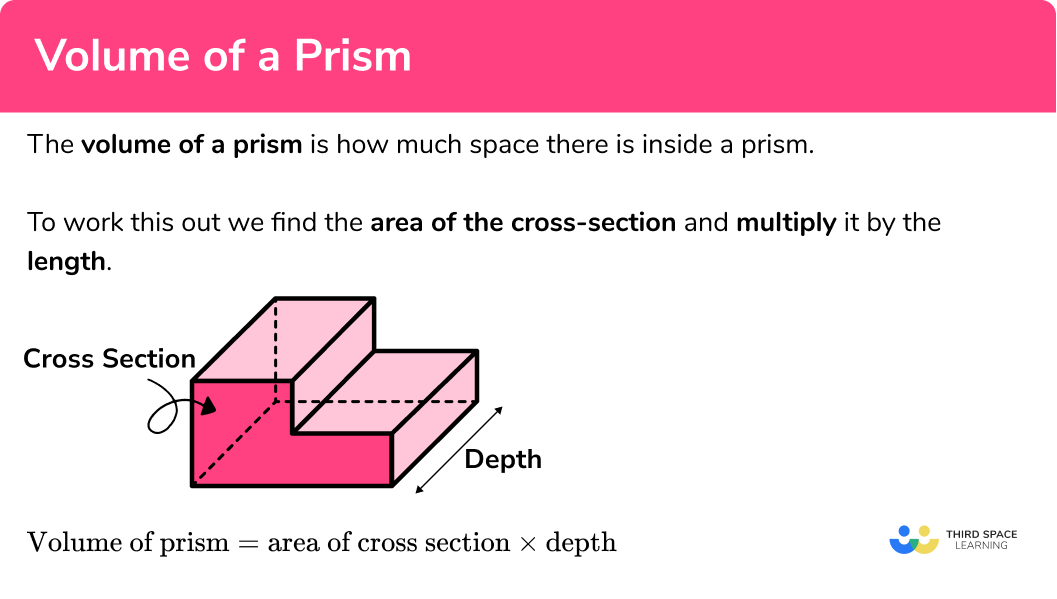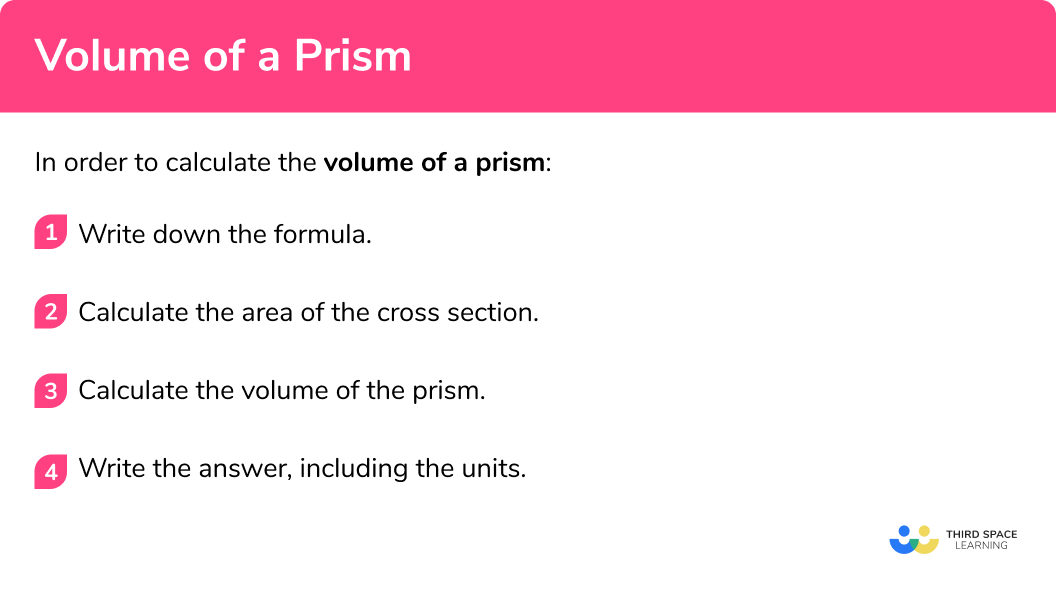# Volume Of A Prism

Here we will learn about the volume of a prism, including how to calculate the volume of a variety of prisms and how to find a missing length given the volume of a prism.

There are also volume and surface area of a prism worksheets based on Edexcel, AQA and OCR exam questions, along with further guidance on where to go next if you’re still stuck.

## What is the volume of a prism?

The volume of a prism is how much space there is inside a prism.

Imagine filling this L-shaped prism fully with water. The total amount of water inside the prism would represent the volume of the prism in cubic units.

To calculate the volume of a prism, we find the area of the cross section and multiply it by the depth.

Volume of prism = Area of cross section x depth

### What is the volume of a prism?## How to calculate the volume of a prism

In order to calculate the volume of a prism:

1. Write down the formula.
2. Calculate the area of the cross section.
3. Calculate the volume of the prism.
4. Write the answer, including the units.

### How to calculate the volume of a prism### Related lessons on prism shape

Volume of a prism is part of our series of lessons to support revision on prism shape. You may find it helpful to start with the main prism shape lesson for a summary of what to expect, or use the step by step guides below for further detail on individual topics. Other lessons in this series include:

## Volume of a prism examples

### Example 1: volume of a triangular prism

Work out the volume of the triangular prism:

1. Write down the formula.

Volume of prism = Area of cross section × depth

2Calculate the area of the cross section.

$\text{Area of triangle }=\frac{1}{2}\times{b}\times{h}\\ =\frac{1}{2}\times{8}\times{3}\\ =12$

The area of the triangle is 12cm^2 .

3Calculate the volume of the prism.

The depth of the prism is 10cm .

$\text{Volume of prism }=\text{Area of cross section }\times\text{depth}\\ =12\times{10}\\ =120$

4Write the answer, including the units.

The measurements on this triangular prism are in centimetres so the volume will be measured in cubic centimetres.

Volume = 120cm^3

### Example 2: volume of a rectangular prism (cuboid)

A swimming pool is being built in the shape of a cuboid.

Calculate the volume of water in the pool when it is completely filled, in litres.

Volume of prism = Area of cross section × depth

$\text{Area of rectangle }=b\times{h}\\ =12\times{3}\\ =36$

The area of the rectangle is 36m^2 .

The depth of the prism is 5m .

$\text{Volume of prism }=\text{Area of cross section }\times\text{depth}\\ =36\times{5}\\ =180$

The measurements on this prism are in metres so the volume will be measured in cubic metres, however we then need to convert this to litres, as it is stated in the question.

Volume = 180m^3 .

1m^3 = 1000L and so 180m^3 180,000L.

The volume of water in the swimming pool in litres is 180,000L .

Note: You may also use the formula:

Volume of cuboid = height × width × depth

since the area of a rectangle is equal to height × width.

### Example 3: volume of a hexagonal prism

Work out the volume of the prism:

Volume of prism = Area of cross section × depth

In this example, we are told that the area of the hexagon is 50mm^2 so we can move on to the next step.

$\text{Volume of prism }=\text{Area of cross section }\times\text{depth}\\ =50\times{15}\\ =750$

The measurements on this prism are in millimetres so the volume will be measured in cubic millimetres.

Volume = 750mm^3

### Example 4: volume of a compound prism

Work out the volume of the L-shaped prism:

Volume of prism = Area of cross section × depth

To calculate the area of the cross section we need to split it into two rectangles and work out the missing side lengths. We can then work out the area of each rectangle:

Rectangle A:

$\text{Area }=7\times{4}\\ =28$

Rectangle B:

$\text{Area }=6\times{5}\\ =30$

Total area:

28+30=58cm^2

The depth of the prism is 12cm .

$\text{Volume of prism }=\text{Area of cross section }\times\text{depth}\\ =58\times{12}\\ =696$

The measurements on this prism are in cm so the volume will be measured in cm^3 .

Volume = 696cm^3

### Example 5: volume of a trapezoidal prism

Work out the volume of the prism:

Volume of prism = Area of cross section × depth

$\text{Area of cross section }=\frac{1}{2}(a+b)h\\ =\frac{1}{2}(2+4) \times 3\\ =9\mathrm{cm}^{2}$

$\text{Volume of prism }= \text{Area of cross section } \times \text{ depth}\\ =9 \times 5\\ =45$

Volume = 45cm^3 .

## How to work out a missing length given the volume

Sometimes we might know the volume and some of the measurements of a prism and we might want to work out the other measurements. We can do this by substituting the values that we know into the formula for the volume of a prism and solving the equation that is formed.

1. Write down the formula.
Volume of a prism = Area of cross section × depth
2. Calculate the area of the cross section.
3. Substitute known values into the formula, and solve the equation.
4. Write the answer, including the units.

## Missing length examples

### Example 6: missing length in a pentagonal prism

The volume of this prism is 225cm^2 . Work out the depth, L, of the prism:

Volume of prism = Area of cross section × depth

In this example, we are told the area of the cross section is 25cm^2

\begin{aligned} \text{Volume of prism }&=\text{Area of cross section }\times \text{depth}\\ 225&=25 \times D\\ 25D&=225\\ D&=9 \end{aligned}

Since the units in this question are in cm and cm^3 , the depth of the prism is 9cm .

### Example 7: missing height in a trapezoidal prism

The volume of this prism is 336cm^3 . Work out the height of the prism.

Volume of prism = Area of cross section × depth

$\text{Area of trapezium }=\frac{1}{2}(a+b)h\\ =\frac{1}{2}(5+9) \times h\\ =7h$

$\text{Volume of prism }=\text{Area of cross section } \times \text{depth}\\ 336=7h \times 8\\ 336=56h\\ 56h=336\\ h=6$

Since the units in this question are in cm and cm^3 , the height of the prism is 6cm .

### Common misconceptions

• Missing/incorrect units

You should always include units in your answer. Remember, volume is measured in units cubed (e.g. mm^3, cm^3, m^3 etc)

• Calculating with different units

You need to make sure all measurements are in the same units before calculating volume. (E.g. you can’t have some in cm and some in m )

• Using the incorrect formula

Be careful to apply the correct prism related formula to the correct question type.

### Practice volume of a prism questions

1. Work out the volume of the prism:192cm^348cm^396cm^340cm^3\text{Area of triangle }=\frac{1}{2} \times 4 \times 6\\ =12\mathrm{cm}^{2}

\text{Volume of triangular prism }=12 \times 8\\ =96\mathrm{cm}^{3}

2. Calculate the volume of the prism:220cm^3308cm^31540cm^3264cm^3\text{Area of trapezium }=\frac{1}{2}(5+7) \times 4\\ =24\mathrm{cm}^{2}

\text{Volume of prism }=24 \times 11\\ =264\mathrm{cm}^{3}

3. Work out the volume of the prism:240cm^32.4cm^32400cm^35760cm^3Area of cross section = 24cm^2

\text{Volume of prism }=24 \times 10\\ =240 \mathrm{cm}^{3}

4. Work out the volume of the prism:84cm^31680cm^31008cm^31344cm^3Area of triangle A :

\text{Area }=\frac{1}{2} \times 7 \times 6\\ =21\mathrm{cm}^{2}

Area of rectangle B :

\text{Area }=7 \times 9\\ =63 \mathrm{cm}^{2}

\text{Total area: } 21+63=84\mathrm{cm}^{2}

\text{Volume of prism }=84 \times 16\\ =1344\mathrm{cm}^{3}

5. The volume of this prism is 156cm^3 .
Work out the depth, x , of the prism.13cm1872cm1.08cm6.5cm\text{Volume of prism} = \text{Area of cross section} \times \text{depth}\\ 156=12x\\ 12x=156\\ x=13

6. The volume of this prism is 270mm^3 . Work out the height, h , of the prism.150mm30mm6mm60750mm\text{Area of parallelogram }=5 \times h \begin{aligned} \text{Volume of prism }&=\text{Area of cross section }\times \text{depth}\\ 270&=5h \times 9\\ 270&=45h\\ 45h&=270\\ h&=6 \end{aligned}

### Volume of a prism GCSE questions

1. Work out the volume of the prism. State the units in your solution.(3 marks)

\text{Area of cross section }=2 \times 4 + 4 \times 1=12\text{cm}^2
or
\text{Area of cross section }=2 \times 3 + 6 \times 1=12\text{cm}^2
For calculating the cross-sectional area of the prism

(1)

\text{Volume of prism: }12 \times 5=60
For calculating the volume of the prism

(1)

60cm^3
For correct units

(1)

2. The volume of the cuboid is twice the volume of the triangular prism. Work out the height, y , of the cuboid.(5 marks)

\frac{1}{2} \times 9 \times 4=18\mathrm{cm}^{2}
For area of the cross section (triangle)

(1)

18 \times 5=90 \mathrm{cm}^{3}
For volume of the triangular prism

(1)

3 \times y \times 6=18y
For volume of the cuboid

(1)

90 \times 2=180\text{ and } 18y=180
Forming an equation to calculate the height of the cuboid

(1)

y=10cm

(1)

3. (a) Calculate the volume of the trapezoidal prism.(b) The prism is made from aluminum, which has a density of 2.7g/cm^3 . Work out the mass of the prism. State the units in your answer.

(4 marks)

(a)
\frac{1}{2}(2+6) \times 4 = 16\mathrm{cm}^{2}
For area of the cross section (trapezium)

(1)

16 \times 8=128 \mathrm{cm}^{3}
For volume of the prism

(1)

(b)
128 \times 2.7
For using Mass = Density \times Volume

(1)

=345.6g
For correct solution including units

(1)

## Learning checklist

You have now learned how to:

• Know and apply formulae to calculate the volume of prisms
• Use the properties of faces, surfaces, edges and vertices to solve problems in 3-D
• Calculate the volume of composite solids

## Still stuck?

Prepare your KS4 students for maths GCSEs success with Third Space Learning. Weekly online one to one GCSE maths revision lessons delivered by expert maths tutors.

Find out more about our GCSE maths tuition programme.